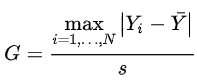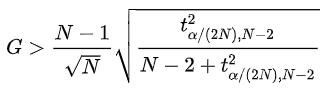# Hypothesis Testing - Grubbs' Test

To understand Grubb's Test, you need to understand what is hypothesis testing in statistics. A hypothesis is an educated guess about something in the world around you. It should be testable, either by experiment or observation. To propose such a hypothesis, you need to put it down in a statement which usually looks like the following:

If I... (do this to an independent variable)... then (this will happen to the dependent variable).

For example, if I stop watering this plant then it will die.

Hypothesis or significance testing is a mathematical model for testing a claim, idea or hypothesis about a parameter of interest in a given population set, using data measured in a sample set.

Here is a simple example: A college reports that students in their school score an average of 8 out of 10 in examinations. To test this "hypothesis," we record marks of say 70 students (sample) from the entire student population of the college (say 1000) and calculate the mean of that sample. We can then compare the (calculated) sample mean to the (reported) population mean and attempt to confirm the hypothesis.

Usually, the reported value (or the claim statistics) is stated as the hypothesis and presumed to be true. For the above examples, the hypothesis will be:

Students in the college score an average of 8 out of 10 in examinations. This stated description constitutes the "Null Hypothesis (H0)" and is assumed to be true until proven otherwise. Whatever information that is against the stated null hypothesis is stated in the Alternative Hypothesis denoted by H1. In this case, the alternative hypothesis will be:

Students score an average that is not equal to 8.

Grubbs' test is defined for the hypothesis:

``````Ho: There are no outliers in the data set
H1: There is exactly one outlier in the data set
``````

So how do we do the hypothesis testing in case of Grubb's test? We calculate a value called ESD as follows:Here `Y-bar` and `s` denotes the sample mean and standard deviation, respectively.

Then we calculate another value called the critical value as shown below:With t*alpha/(2N),N-2 denoting the critical value of the t distribution with (N-2) degrees of freedom and a significance level of alpha/(2N).

In this case, if the critical value is greater than the calculated value, we accept the null hypothesis and conclude that there are no outliers and vice versa.

Now, let's put this into work using Python.

INSTRUCTIONS
• Import Numpy as `np` and `scipy.stats` as `stats`

``````import numpy as <<your code goes here>>
import scipy.stats as <<your code goes here>>
``````
• Define the data using 2 arrays, `x` and `y` as follows

``````x = np.array([12,13,14,19,21,23])
<<your code goes here>> = np.array([12,13,14,19,21,23,45])
``````
• Now let's define a function `grubbs_test` to put the above theory into pratice

``````def <<your code goes here>>(x):
n = len(x)
mean_x = np.mean(x)
sd_x = np.std(x)
numerator = max(abs(x-mean_x))
g_calculated = numerator/sd_x
print("Grubbs Calculated Value:",g_calculated)
t_value = stats.t.ppf(1 - 0.05 / (2 * n), n - 2)
g_critical = ((n - 1) * np.sqrt(np.square(t_value))) / (np.sqrt(n) * np.sqrt(n - 2 + np.square(t_value)))
print("Grubbs Critical Value:",g_critical)
if g_critical > g_calculated:
print("Using Grubbs' test we observe that calculated value is lesser than critical value. So we accept null hypothesis and conclude that there are no outliers\n")
else:
print("Using Grubbs' test we observe that calculated value is greater than critical value. So we reject null hypothesis and conclude that there is atleast one outlier\n")
``````
• Finally, let's test this function on `x` and `y` by running the following codes in 2 different cells

``````grubbs_test(x)

grubbs_test(y)
``````

No hints are availble for this assesment

Answer is not availble for this assesment

Note - Having trouble with the assessment engine? Follow the steps listed here